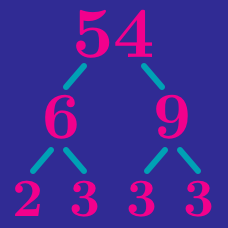Number Theory

# Prime Factorization

$72 = 8 \times 9$

What is the largest prime factor of $72$?

Which of these sets lists all of the prime factors of $120$?

How many distinct prime factors does $2^{8}-1$ have?

Hint: You can use the difference of squares formula to help factor.

What is the sum of all the distinct prime factors of $495$?

Details and assumptions

Only add the prime factor once. For example, the sum of the prime factors of $45 = 3^2\times 5$ is $3 + 5 = 8$, not $3 + 3 + 5 = 11$.

Which of these numbers has more distinct prime factors?

$45 \quad 56$

×